Heart Curve
 To the Main Page    "Mathematische Basteleien"

What is the Heart Curve?The heart curve is a closed curve, which has the shape of a heart.  The heart is well known as a figure on playings cards besides diamonds, cross and spades.

If you speak about a heart, you rather mean the heart figure than the heart shaped curve.

 ...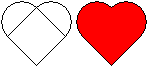... In the simplest case, a heart is formed by a square standing on its point and two semi-circles sitting on the sides. Characteristics of the heart figure obviously are a groove above and a point below.

 ...... A heart figure develops also if you set two semi-circles upon a triangle. But here you get two unpleasant corners. Obviously you expect that these sides are rounded.

 ...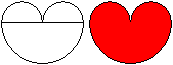... If the point below is missing then don't speak of a heart but rather of a heart shaped  figure. This form, however, is more similar to the human heart.  The figure on the left is formed by three semi-circles.

Drawn Heart Curves top
Method 1
 ...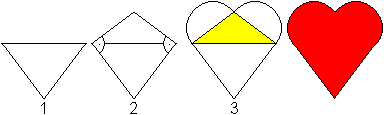... 1 Draw an isosceles triangle. 2 Draw the perpendiculars to the legs.  They produce a second isosceles triangle.  3 Draw two semi-circles upon the legs of the (now yellow) triangle.

Method 2
 ...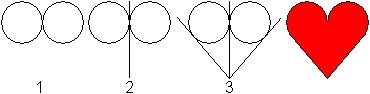... 1 Draw two touching equal circles.  2 Draw the common tangent. 3 Draw two further (outer) tangents from one point of the  tangent.

Method 3
 ...... 1 Draw a square.  2 Draw four equal circles. The centres are the corners of the square and the common radius is "half square side".

Method 4
 ...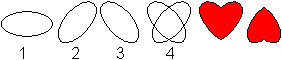... 1 Draw an ellipse. 2 Turn it about 45°. 3 Reflect it. 4 Form two hearts.

Method 5
 ...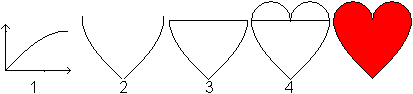... 1 Draw the graph of f(x)=sin(x), 0

Method 6
 ...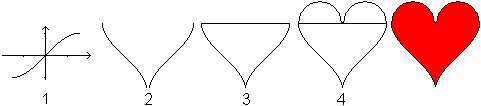... 1 Draw the graph of f(x)=sin(x), -pi/2

Calculated Heart Curves     top
It is a challenge to find formulas which produce hearts.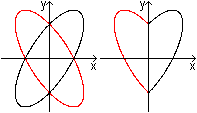You can describe method 4 by formulas. The black ellipse has the formula 2x²-2xy+y²-1=0.The domain is {x| x>=0}. The red ellipse has the formula 2x²+2xy+y²-1=0. The domain is {x| x<=0}.
You can leave out the domain, if you isolate y and use the function f(x)=|x|.
Then y=|x|+sqrt(1-x²) and y=|x|-sqrt(1-x²) describe a heart curve [(7)].

The formulas y=sqr(|x|)+sqrt(1-x²) /\ y=sqrt(|x|)-sqrt(1-x²) are even better.

More curves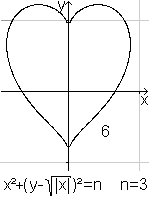Sources:
(Picture 1) Book 8, Eugen Beutel (1901) (2) Buch 4, Aufgabe 8.5.5., (3) MathWorld (simplified formulas), (4) H.-J. Caspar's web site   (URL below), (6) Jurjen N.E. Bos

From the desk of Torsten Sillke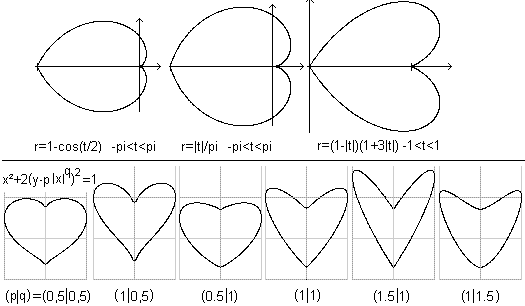Threedimensional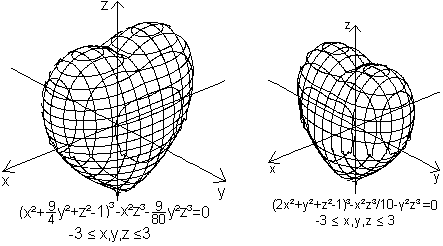If you choose y=0 respectively x=0, you get the equation of the 2D heart above on the left.

Source: Gabriel Taubin [for example MathWorld (URL below)]

The graphs were made by the freeware program "winplot" (Version 23.05.2000, URL below).

Winword Hearts top
And how do artists design a heart?

The heart appears as a well known figure in character sets of programs under MS Windows. There it is a figure of  playings cards besides diamonds, cross and spades.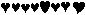Here is a choice of  well known character sets.

The sets are Normaler Text, Arial, Courier New, Estrangelo Edessa, Lucida Console, Symbol, Times New Roman, Webdings.
If you increase the letters from 12 to 72 you recognize the shapes.The originally black colour is replaced by the heart colour red.

The upper part of the heart figure is formed by curves similar to arcs.  The lower lines do not approach linearly to the point but usually are at first inward and then outward curved.  That gives the heart a special sweep.

Found at Unicode Standard, Version 4.01 BLACK HEART SUIT 2665  2 WHITE HEART SUIT 2664 3 HEAVY BLACK HEART 2764

Pupils' Hearts top
And how do students draw a heart?

23 pupils of HS Lohfeld at Bad Salzuflen (Germany) were to draw a simple heart.
The results are:Thanks to Klasse 7a,  Jg.2003/2004, about 12 years old.

Heart Curve or Cardioid top
How to produce it
 ...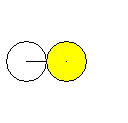... Draw a circle (on the left, yellow) and roll an equal circle on it.  Fix one point on the moving circle line and follow this point. It describes the heart curve or cardioid (on the right). ...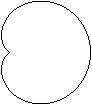...

Another way of producing a heart
 ...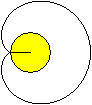... A cardioid can also be seen as the envelope of circles.  Draw a (yellow) circle and a fixed point P on the circle line.  All circles that pass through the fixed point P and have their centres on the (yellow) circle have a cardioid as an envelope.

Area and perimeter of the heart curve
Use the polar form  r=2a[1+cos (t)] as the simplest equation for calculating the area A and the perimeter U. The origin of a coordinate system lies in the point of the cardioid.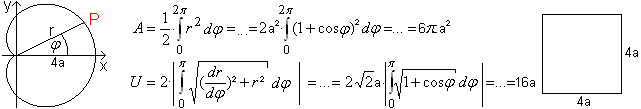The perimeter is a rational number. A square with the side 4a has the same one.

Mandelbrot Set and Cardioid
 ...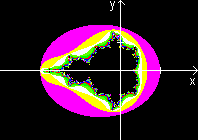... The "main figure" of the Mandelbrot set has the form of the cardioid. The German name Apfelmännchen (apple man) uses this shape.  Actually the main figure is a cardioid. The points of the Mandelbrot set, which have convergent sequences, lie inside a cardioid.  Source: (5), page 208ff. There you find a proof and more references.
The picture was taken from my page Mandelbrot Set

Catacaustic and Cardioid
 ...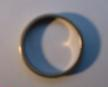... If light is falling on a spheric mirror (wedding ring in the sun light), the reflecting rays form a special surface, the catacaustic. It isn't a cardioid but a nephroid. A cardioid develops as an envelope, if the rays start at a point on the circle and are reflected at the circle (drawing on the right). ...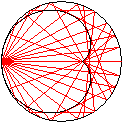...
You find more on my German page Ringe.

Characteristic Curve of a Microphone
 ...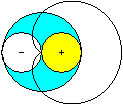... Microphones have a certain characteristic curve. In the plane it is a circle for the "sound-pressure-receiver" and similar to a lying eight figure for the "sound-velocity-receiver". Special receivers like condenser microphones have both capacities. Their characteristic curve develops by overlaying to a cardioid.
Source: (6), page 550

The Broken Heart top
The broken heart (Das gebrochene Herz) is a tangram game.
 ...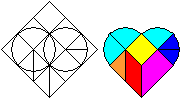... Lay inside a square two circles and draw some lines. A heart develops, which is divided in nine pieces. The fun is to lay a heart with the pieces or to discover new figures like those on the right. ......
Source: (1) page 22, (2) page 140-145

The Woven Heart top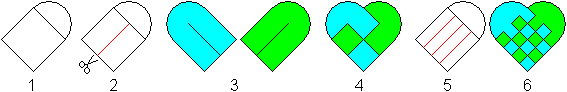1 Draw a square and two half circles sitting on it.
2 Cut it in along the red line.
3 Copy it.  Paint the paper in two different colours or start with coloured paper.
4 Insert the blue piece in the green piece.
5,6 The heart works fine with a larger number of slits as well.

 ...... If you like have a look at the heart basket page made by Christopher Hamkins.

Tesselation of Hearts  top
 ...... 1 Give a spiral. 2 Reflect the spiral on the end point. 3 Connect both spirals to get a double spiral. 4 Reflect the double spiral. It forms a heart together with the first double spiral. Many hearts lead to a tesselation (on the right). ...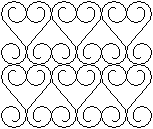...
The idea for this drawing came from a window grill in Venice (June 2004):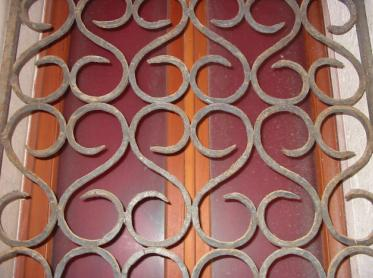Venice is rich with heart grills.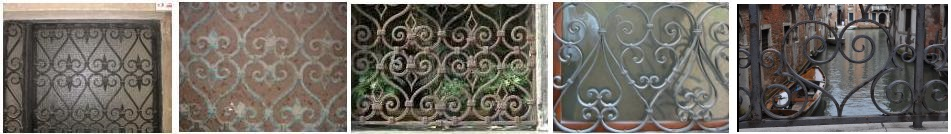Rosettes of Heart Figures topfour-leaf clover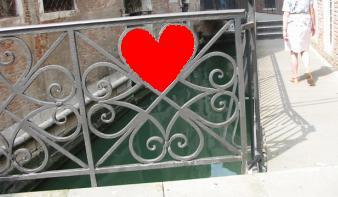Once again a Venice photo- brightened up with some red.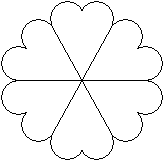Six wafflesA pair of swans during the courtship display
Photo on the web site fotocommunity (URL below)

Emoticons   topReferences   top
(1) Pieter van Delft, Jack Botermans: Denkspiele der Welt, München 1998 ISBN 3-88034-87-0]

(2) Karl-Heinz Koch: ...lege Spiele, Köln 1987 (dumont taschenbuch1480)  [ISBN 3-7701-2097-3]

(3) Heinz Nickel u.a: Algebra und Geometrie für Ingenieur- und Fachschulen, Frankfurt / Zürich 1966

(4) Hans Schupp, Heinz Dabrock: Höhere Kurven,  BI Wissenschaftsverlag 1995 [ISBN 3-411-17221-5]
x^2 + 2( 3/5 (x^2)^(1/3) - y )^2 = 1

(5) Herbert Zeitler: Über die Hauptkörper spezieller Funktionen, MNU, Jg.52, 1999, Heft4

(6) Bergmann-Schaefer: Lehrbuch der Expermentalphysik, Berlin, NewYork 1975 [ISBN 3 11 004861 2]

(7) Norbert Herrmann: Mathematik ist überall, Oldenbourg Verlag 2004 [ISBN 3-486-57583-X]
y = |x| +- sqrt(1 - x^2)
y = 2/3 ( (x^2 + |x| - 6)/(x^2 + |x| + 2) +- sqrt(36 - x^2) ) (siehe auch Webseite von Thomas Jahre)

(8) Eugen Beutel: Algebraische Kurven, G.J. Göschen, Leipzig 1909-11
(x^2 + y^2 - 1)^3 = 4x^2y^3

(9) Ulrich Graf: Kabarett der Mathematik,  Dresden: L. Ehlermann, 1942 Hardcover, 1.Auflage. (1943 Hardcover. 2. Auflage.)

(10) Michael Zettler: Und noch ein Herz. PM 6/99 Seite 274
y = sqrt(1 - (|x|-1)^2),  y = arccos(1 - |x|) - pi

(11) Thomas Hechinger:   ... und noch ein weiteres Herz. PM 2/00 Seite 67
y = sqrt(1 - (|x|-1)^2),  y = -3 sqrt(1 - sqrt(|x|/2))

(12) Mitteilung von  Torsten Sillke:
x^2 + 2 (y - p*|x|^q)^2 = 1 (siehe Schupp / Eisemann)
r = 2 sin^2(phi/4) = 1 - cos(phi/2) mit |phi| <= pi  (nach Eisemann)
r = |phi|/pi                                     mit |phi| <= pi. (Archimedische Spirale)
r = (1 - |phi|)(1 + 3|phi|)                mit |phi| <= 1. (nach Caspar)

(13) El-Milick, Elements d'Algebre Ornementale, Paris, 1936:y=(x)^(2/3)+(a²-x²)^(1/2) und y=x^(2/3)-(a²-x²)^(1/2) and a=2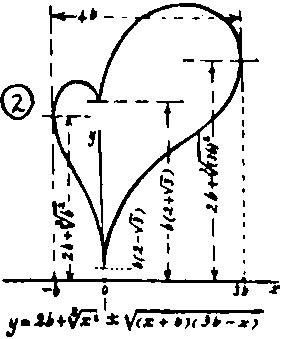Heart Curves on the Internet top

German

33. Evangelischer Kirchentag in Dresden 2011

Armin Dietz
Das Herzsymbol

Christian Ucke und Christian Engelhardt
Kaustik in der Kaffeetasse
[erschienen in: Physik in unserer Zeit, 29 (1998), Seite 120 bis 122]
 Benutzer:Georg-Johann (Wikipedia) Von Punkt zu Punkt x(t)=12sin(t)-4sin(3t) y(t)=13cos(t)-5cos(2t)-2cos(3t)-cos(4t)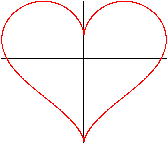luther.de
Die Lutherrose
 Ernst-Moritz-Arndt-Universität Greifswald Herzkartenentwurf von Stabius-Werner (Mathematik und Kunst) Dies ist die Formel des Randes des "Herzkartenentwurf von Stabius-Werner" X =        t sin( 3.14 sin(t)/t ) Y = - abs(t) cos( 3.14 sin(t)/t ) -pi <= t <= pi (Mitteilung von Torsten Sillke)
Fotocommunity
Frühlingsgefühle  (Thomas Th.)

Hans-Jürgen (Matroids Matheplanet)
Herzkurven

Hans-Jürgen Caspar
Kurven
x = a (-phi² + 40 phi +1200) sin(pi*phi/180)
y = a (-phi² + 40 phi +1200) cos(pi*phi/180)

Jan Schormann
u.a. Bézierkurven

Friedrich Krause
y = sqrt(|x|) +- sqrt(1 - x^2)
 Klaus Rohwer Fraktale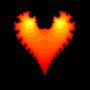Michael Holzapfel
Herzkurve aus zwei Funktionsteilen
y = sqrt(1 - (|x|-1)^2),  y = arccos(1 - |x|) - pi
(x^2 + y^2)(x^2 + y^2 - 2ax) - a^2y^2  =  0 (Kardioide)

NN (Matheplanet)
Geometrie in der Teetasse

 Thomas Jahre (Chemnitzer Schulmodell) Ein Herz für die Mathematik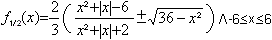Torsten Sillke
Herzkurven

Wikipedia
Kardioide, Herz (Symbol), Herz (Heraldik), Herz (Farbe)

English

Alex Bogomolny (Cut The Knot!)
Hearty Munching on Cardioids

Eric W. Weisstein (MathWorld)
 Cardioid Heart Curve (x²+y²-1)³-x²y³ (siehe Beutel) x=sin(t)cos(t)ln|t| ; y=|t|0.3[cos(t)]0.5 mit 0<=t<=1 Bonne Projection Heart Surface [x²+(9/4)y²+z²]³-x²z³-(8/90)y²z³=0 (2x²+2y²+z²-1)³-(1/10)x²z³-y²z³=0
Circle Catacaustic

Four Ways to 3D Hearts: Part 1

Jan Wassenaar
cardioid

JOC/EFR (School of Mathematics and Statistics, University of St Andrews, Scotland)
Cardioid

Kurt Eisemann
x^2 + (y - 3/4 (x^2)^(1/3))^2 = 1 (Footnote)
r = sin^2( pi/8 - phi/4 ) (Footnote)

pacifict.com
Heart  (3D)

Pavel Boytchev
Mathematics ... loves you   (Video)

Richard Parris (Freeware-Programme)
winplot

Wikipedia
Cardioid, Heart (symbol)

Xah Lee
Cardioid

French

Robert FERRÉOL (mathcurve)
CARDIOIDE, DOUBLE-COEUR
 Coeur de Raphaël Laporte x=sin3 t y=cos t -sin4 t

Japanese

Nobuo YAMAMOTO
Heart CurvesHeart Curves IIHeart Curves IIb

Thanks to Torsten Sillke for several hints.
Gail from Oregon Coast - thank you for supporting me in my translation.

Found on the Internet ;-):
the nerdy way of drawing a heart.
http://www.mathematische-basteleien.de/heart.htm

Must they do EVERYTHING in math? ><;; lol.

Feedback: Email address on my main page